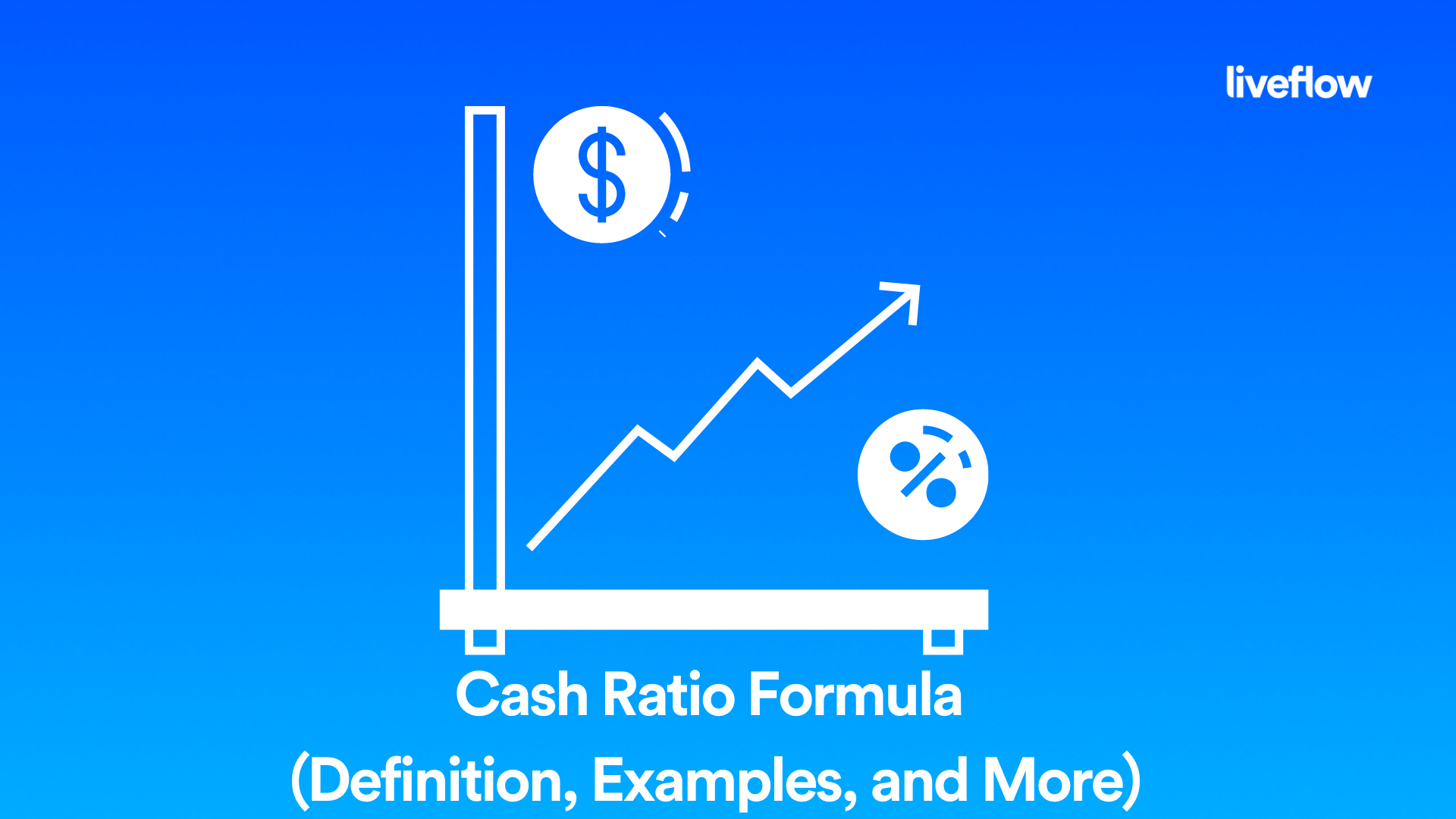# Cash Ratio Formula (Definition, Examples, and More)

July 27, 2022When it comes time to calculate your balance sheet, you may need to use the cash ratio formula. This is a common liquidity formula that businesses use to determine how much of their money is liquid and how much is tied to debts and other obligations. But how do you calculate the cash ratio formula, and how is this equation used by business accountants?

In this article we will explain everything you need to know about the cash ratio formula, including how it’s calculated and when to use it.

## What is meant by cash ratio?

Cash ratio is one of the financial ratios used in business accounting to indicate how effectively a company manages its cash assets. It is calculated by dividing the amount of cash and marketable securities by current liabilities. The ratio indicates how much of the current liabilities are paid with cash and marketable securities, while the rest is financed by debt or other sources.

## What is a good cash ratio?

The cash ratio indicates how easily a business can meet its current obligations. If it's lower than 1, it's a sign that the company may not be able to pay off its debt. However, if it's higher than 1, it doesn't necessarily mean that the business is financially healthy. A higher cash ratio could also mean that the company is hoarding cash and not investing in growth opportunities.

In general, it's better for a company to have a low cash ratio (close to 1) than a high one (above 1). But there are exceptions — for example, companies with very stable earnings might have high ratios because they don't need to use their cash for daily operations or expansion plans.

## How do you calculate cash ratio?

If you’re wondering how to calculate cash ratio it is actually relatively simple, you simply need to use the cash ratio formula. By using this simple equation, you will be able to determine the cash ratio of a business at any given time and take appropriate action to adjust your balance sheet accordingly. Let’s briefly go over what the cash ratio formula is and how to calculate cash ratio.

## What is the cash ratio formula?

The cash ratio formula is calculated as follows:

Cash Ratio = Cash + Marketable Securities / Current Liabilities

By using this simple calculation, you will be able to determine the cash ratio of your business and make any necessary adjustments to keep your business on budget and profitable without having to liquidate any assets or take on additionally credit facilities in order to continue operating.

## What is cash ratio with example?

Cash ratio is the ratio of cash and cash equivalents to current liabilities. In other words, cash ratio is a measure of liquidity and a measure of a company's ability to pay off its short-term debt obligations.

Companies with high levels of cash can afford to take on more risk because they have extra resources if their investments don't pan out as well as expected. Companies that have little cash may be forced to sell assets quickly if business conditions worsen.

## How do you calculate cash ratio from balance sheet?

Cash ratio is the ratio of cash and equivalents to current liabilities. It indicates how much the company can pay its short-term obligations with cash and cash equivalents on hand.

The following three steps will show you how to calculate cash ratio from your balance sheet:

1. Find Cash and Equivalents in Assets section of Balance Sheet

2. Find Current Liabilities in Liabilities section of Balance Sheet

3. Divide Cash and Equivalents by Current Liabilities to get Cash Ratio

That’s about all there is to it, the cash ratio formula is one of the easiest financial ratios to calculate but it is also important not to overlook it when filling out your balance sheet.

## Summary

Cash ratio is the amount of cash on hand divided by total current liabilities. This is an important liquidity ratio because it shows how much cash a company has relative to its short-term obligations. A higher ratio indicates more liquidity, while a lower ratio indicates less liquidity.

## Recommendation

One of the best financial accounting tools on the market, LiveFlow has helpful templates that make accounting easier than ever. So, If you want to simplify your financial accounting and make the most of your data, then be sure to check out LiveFlow today. Best of all, you can explore all of the best features with a free 30-minute demo, so you have nothing to lose and everything to gain.